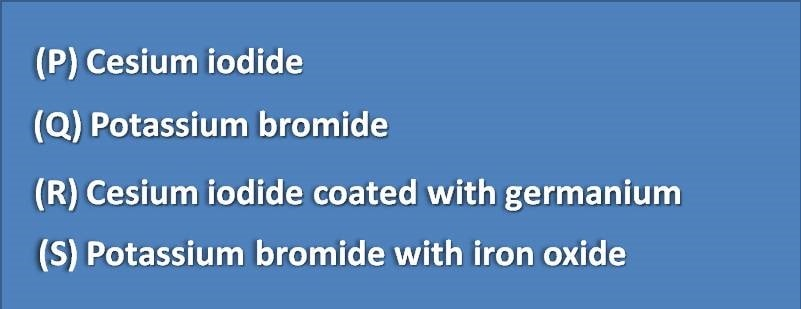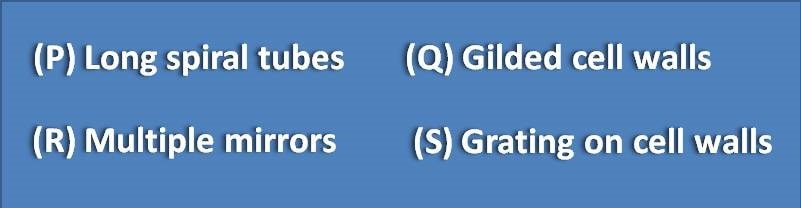# MCQ on IR spectroscopy: Page-7

1.Interferogram is plot of

(A) Volts vs time

(B) Volts vs concentration

(C) Volts vs wavelength

(D) Volts vs Wave number

2. Interferogram is produced as a function of

(A) Change of Intensity of between two beams

(B) Change of path length between two beams

(C) Change of energy between two beams

(D) All of the above

3. Beam splitters in IR spectroscopy are made up of(A) Q,S

(B) P,R

(C) R,S

(D) P,Q

4. Which of the following type of samples require multiple path length for a significant absorption in IR.

(A) Solid samples

(B) Liquid samples

(C) Gaseous samples

(D) All the above

Multiple path lengths in IR absorption analysis can be achieved by(A) Q,S

(B) P,R

(C) P,S

(D) Q,R